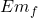## If you slide down a rope, it’s possible to create enough thermal energy to burn your hands or your legs where they grip the rope. Suppose a

Question

If you slide down a rope, it’s possible to create enough thermal energy to burn your hands or your legs where they grip the rope. Suppose a 30 kg child slides down a rope at a playground, descending 2.5 m at a constant speed.
How much thermal energy is created as she slides down the rope?

in progress 0
5 months 2021-08-25T11:40:00+00:00 1 Answers 12 views 0

Q = 735 J

Explanation:

In this exercise we must assume that all the mechanical energy of the system transforms into cemite energy.

Initial energy

Em₀ = U = m g h

final energy= Q

Em₀ = Em_{f}

m g h = Q

let’s calculate

Q = 30  9.8  2.5

Q = 735 J